# AVERAGEIF

The AVERAGEIF function in Excel calculates the average of cells that meet one criteria. AVERAGEIFS calculates the average of cells that meet multiple criteria.

1. For example, the AVERAGEIF function below (two arguments) calculates the average of all values in the range A1:A7 that are greater than 0.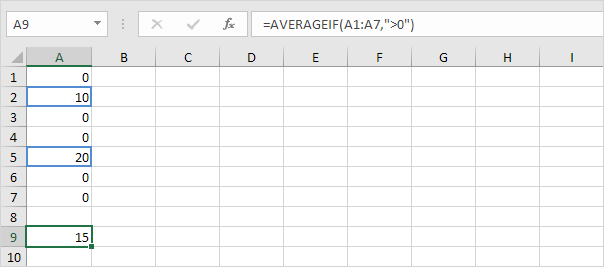2. The AVERAGEIF function below (three arguments, last argument is the range to average) calculates the average of all values in the range B1:B7 if the corresponding cells in the range A1:A7 contain exactly Apple.3. The AVERAGEIF function below calculates the average of all values in the range B1:B7 if the corresponding cells in the range A1:A7 do not contain exactly Banana.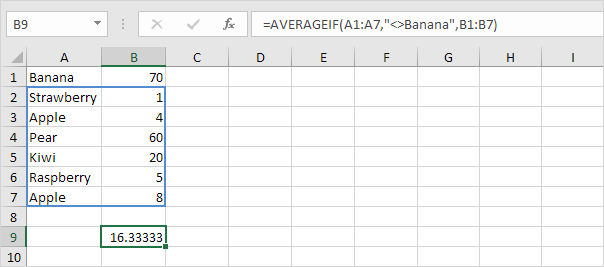4. The AVERAGEIF function below calculates the average of all values in the range B1:B7 if the corresponding cells in the range A1:A7 contain a series of zero or more characters + berry. An asterisk (*) matches a series of zero or more characters.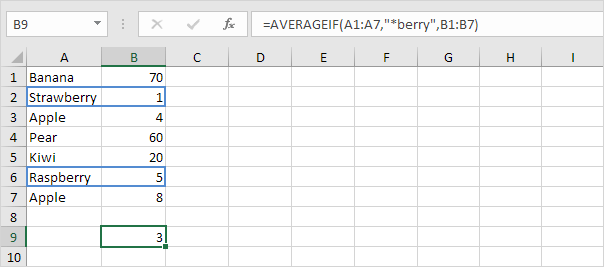5. The AVERAGEIF function below calculates the average of all values in the range B1:B7 if the corresponding cells in the range A1:A7 contain exactly 4 characters. A question mark (?) matches exactly one character.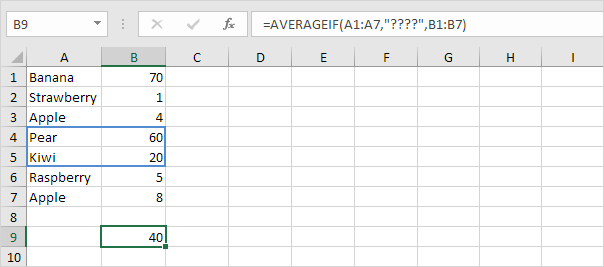The AVERAGEIFS function (with the letter S at the end) in Excel calculates the average of cells that meet multiple criteria.

6. The AVERAGEIFS function below calculates the average of all values in the range A1:A7 that are greater than or equal to 500 and less than or equal to 1000.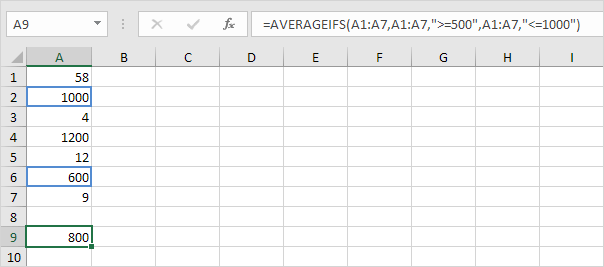Note: first argument is the range to average, followed by two or more range/criteria pairs.

7. The AVERAGEIFS function below calculates the average of all values in the range C1:C7 if the corresponding cells in the range A1:A7 contain exactly Apple and the corresponding cells in the range B1:B7 contain exactly Red.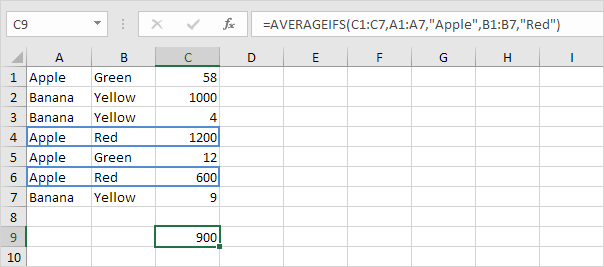Note: again, the first argument is the range to average, followed by two or more range/criteria pairs.

Go to Next Chapter: Round